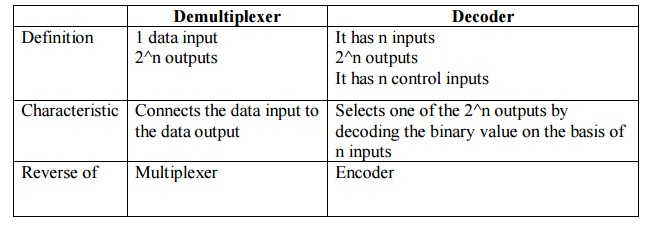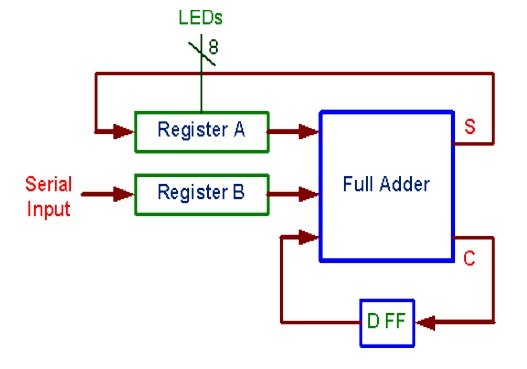Home | | Digital Electronics | Important Short Questions and Answers: Digital Electronics - Combinational Circuits

# Important Short Questions and Answers: Digital Electronics - Combinational Circuits

Digital Electronics - Combinational Circuits - Important Short Questions and Answers: Digital Electronics - Combinational Circuits

COMBINATIONAL CIRCUITS

1             Write an expression for borrow and difference in a full subtractor circuit.

Difference=AB+AB′=AB Borrow=AB

2             Draw the circuits diagram for 4-bit odd parity generator.3. Design a single bit magnitude comparator to compare two words A and B.4             What is an encoder?

An encoder has 2n input lines and n output lines. In encoder the output lines generate the binary code corresponding to the input value.

5             List few applications of multiplexer.

·              Data Selector.

·              Implement combinational logic circuit.

·              Time multiplexing systems

·              Frequency multiplexing systems.

·              D/A and A/D converter

·              Data acquisition systems.

6     Design a half subtractor using basic gates.7     Draw the logic diagram of a 4 line to 1 line multiplexer.8            What is priority Encoder?

A priority encoder is an encoder circuit that includes the priority function. In priority encoder, if 2 or more inputs are equal to 1 at the same time, the input having the highest priority will take precedence.

9     Write down the difference between demultiplexer and decoder.10        Give the logic expression for sum and carry in full adder circuit.

Sum= (AB)Cin Carry=AB+BCin+A Cin

11        Give examples for combinational circuit.

ii.           Subtractors

iii.        Multiplexers

iv.        Demultiplexers

v.           Encoders

vi.        Decoders

12        Draw the logic circuit of a 2-bit comparator.13        Suggest a solution to overcome the limitation on the speed of an adder.

It is possible to increase speed of adder by eliminating inter-stage carry delay. This method utilizes logic gates to look at the lower-order bits of the augend and addend to see if a higher-order carry is to be generated.

14        Relate carry generate, Carry propagate, Sum and Carry-out of a Carry look a head adder.15 Realize the Boolean function using appropriate multiplexer F(A,B,C)= Σ (0,1,3,7)16 Compare the performance of binary serial and parallel adders.

·              Serial adder uses shift registers

·              The serial adder is a sequential circuit

·              Time required for addition depends on the number of bits

·              It is slower

·              It is faster

·              Time required for addition does not depend on number of bits

·              Excluding the registers, the parallel adder is a purely combinational circuit

17       Design of three bit parity generator.

Odd parity generator:18 Draw the logic diagram of serial adder.19       Construct a two-4-bit parallel adder/subtractor using Full Adders and XOR gates.20       Convert a two-to-four line decoder with enable input to 1X4 Demultiplexer.Study Material, Lecturing Notes, Assignment, Reference, Wiki description explanation, brief detail
Digital Electronics : Combinational Circuits : Important Short Questions and Answers: Digital Electronics - Combinational Circuits |